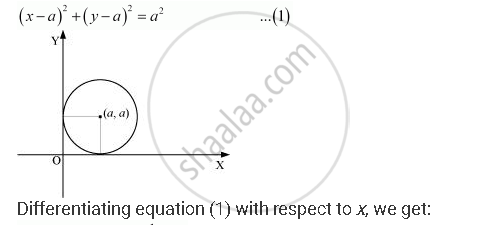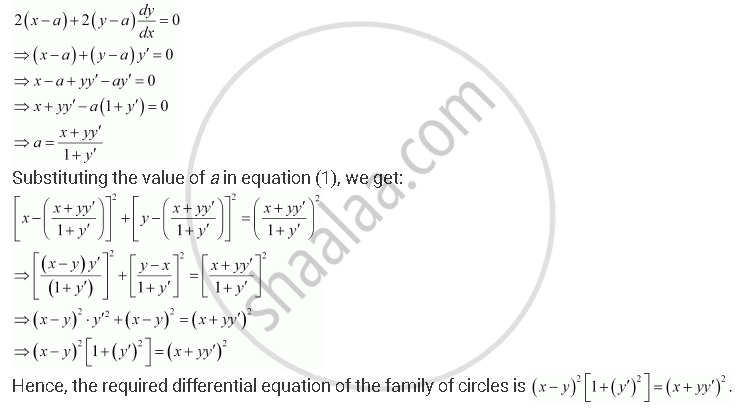Share

# Form the Differential Equation of the Family of Circles in the First Quadrant Which Touch the Coordinate Axes. - CBSE (Science) Class 12 - Mathematics

ConceptProcedure to Form a Differential Equation that Will Represent a Given Family of Curves

#### Question

Form the differential equation of the family of circles in the first quadrant which touch the coordinate axes.

#### Solution

The equation of a circle in the first quadrant with centre (aa) and radius (a) which touches the coordinate axes is:Is there an error in this question or solution?

#### APPEARS IN

NCERT Solution for Mathematics Textbook for Class 12 (2018 to Current)
Chapter 9: Differential Equations
Q: 4 | Page no. 420
Solution Form the Differential Equation of the Family of Circles in the First Quadrant Which Touch the Coordinate Axes. Concept: Procedure to Form a Differential Equation that Will Represent a Given Family of Curves.
S